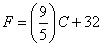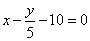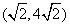# CBSE Important Questions

## Mathmatics Class IX

Q. 1. Solve the equation 2x + 1 = x – 2 & represent the solution on :

1. The number line
2. Cartesian plane

Q. 2. The linear equation that converts the Fahrenheit to Celsius is as follows :-1. Draw the graph of the linear equation .
2. If the temperature is 950F , what is the temperature in Celsius .
3.  Is  there  a  temperature   which   is  numerically  the  same   in   both Fahrenheit and  Celsius ? If yes, find it.

Q. 3. Express the following information in the form of linear equation :

1. In a one day International cricket match between India & Srilanka played in Nagpur, two Indian batsman together scored 176 runs .
2. The cost of a notebook is twice the cost of a pen.

Q. 4. Find two solutions for each of the following equations :

1. 4x + 3y = 12
2.  2x + 5y = 0
3. px + y = 3

Q. 5. Express each of the following linear equation in the standard form & hence find the values of a, b & c in each case :

1.2. 2x + 3y =(c) 2x = -5y

Q. 6. Check which of the following are solutions of the equation x – 2y = 4.

1.2.  (1,1)
3. (4,0)

Q. 7. (a). How many solutions does the following linear equation & why ?

3x – y + 5 = 0
Find the value of k, if x = 2, y = 1 is a solution of the equation 2x + 3y = k.

Q. 8. (a). Given the  point  (1,2),  find  the  equation of a line on which it lies. How many such equations are there ?

Give an equation of two lines passing through (2,14). How many more Such lines are there & why ?

Q. 9. Draw the graph of each of the following linear equation in two variables :

1. 3 = 2x + y
2. y = 3x

Q. 10. The taxi fare in the city is as follows : For the first kilometer, the are is Rs. 8 & for the  subsequent  distance  it  is  Rs. 5  per  km.  Write a  linear equation for this information & draw its graph .